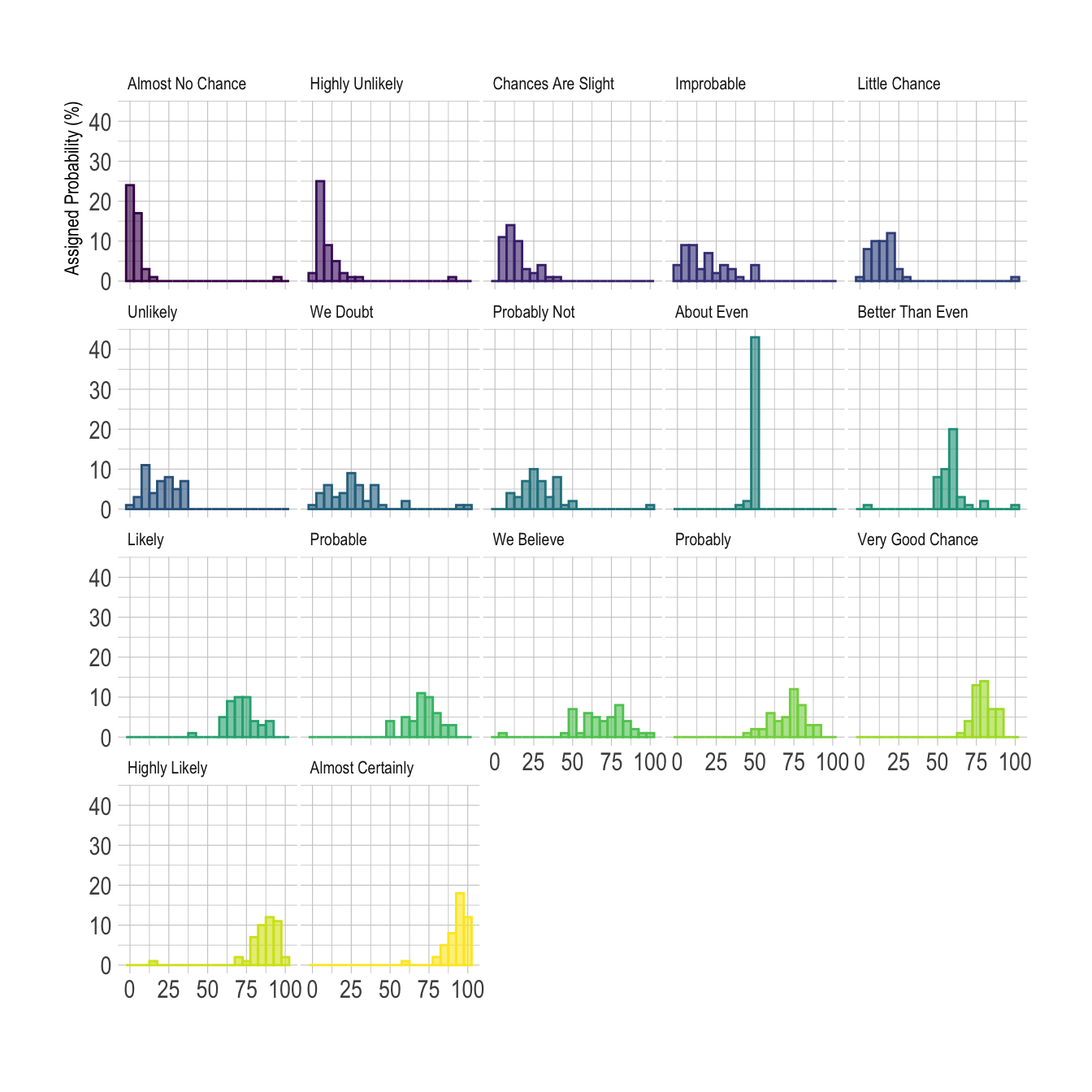# Histogram with several groups - ggplot2

A histogram displays the distribution of a numeric variable. A common task is to compare this distribution through several groups. This document explains how to do so using R and ggplot2.

# Several histograms on the same axis

If the number of group or variable you have is relatively low, you can display all of them on the same axis, using a bit of transparency to make sure you do not hide any data.

Note: with 2 groups, you can also build a mirror histogram

``````# library
library(ggplot2)
library(dplyr)
library(hrbrthemes)

# Build dataset with different distributions
data <- data.frame(
type = c( rep("variable 1", 1000), rep("variable 2", 1000) ),
value = c( rnorm(1000), rnorm(1000, mean=4) )
)

# Represent it
p <- data %>%
ggplot( aes(x=value, fill=type)) +
geom_histogram( color="#e9ecef", alpha=0.6, position = 'identity') +
scale_fill_manual(values=c("#69b3a2", "#404080")) +
theme_ipsum() +
labs(fill="")``````# Using small multiple

If the number of group you need to represent is high, drawing them on the same axis often results in a cluttered and unreadable figure.

A good workaroung is to use small multiple where each group is represented in a fraction of the plot window, making the figure easy to read. This is pretty easy to build thanks to the `facet_wrap()` function of ggplot2.

``````# Libraries
library(tidyverse)
library(hrbrthemes)
library(viridis)
library(forcats)

data <- data %>%
gather(key="text", value="value") %>%
mutate(text = gsub("\\.", " ",text)) %>%
mutate(value = round(as.numeric(value),0))

# plot
p <- data %>%
mutate(text = fct_reorder(text, value)) %>%
ggplot( aes(x=value, color=text, fill=text)) +
geom_histogram(alpha=0.6, binwidth = 5) +
scale_fill_viridis(discrete=TRUE) +
scale_color_viridis(discrete=TRUE) +
theme_ipsum() +
theme(
legend.position="none",
panel.spacing = unit(0.1, "lines"),
strip.text.x = element_text(size = 8)
) +
xlab("") +
ylab("Assigned Probability (%)") +
facet_wrap(~text)``````Related chart types

## Contact

This document is a work by Yan Holtz. Any feedback is highly encouraged. You can fill an issue on Github, drop me a message on Twitter, or send an email pasting yan.holtz.data with gmail.com.